# AP Calculus AB Practice Test 43

### Test Information9 questions18 minutes

Calculator Disallowed

1.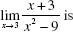2. The only function that does not satisfy the Mean Value Theorem on the interval specifiedis

3. Suppose f (x) = x(x - 2)2(x + 3). Which of the following is (are) true?

I. f has a local maximum at x = -3.

II. f has a local minimum at x = 0.

III. f has neither a local maximum nor a local minimum at x = 2.

4. If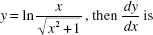5.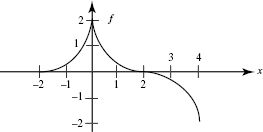The graph of function f shown above consists of three quarter-circles.

Which of the following is (are) equivalent to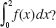I.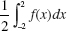II.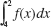III.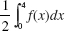6. The base of a solid is the first-quadrant region bounded by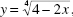and each cross section perpendicular to the x-axis is a semicircle with a diameter in the xy-plane. The volume of the solid is

7. The average value of f (x) = 3 + |x| on the interval [-2, 4] is

8.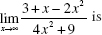9. The area of the region in the xy-plane bounded by the curves y = ex, y = e-x, and x = 1 is equal to